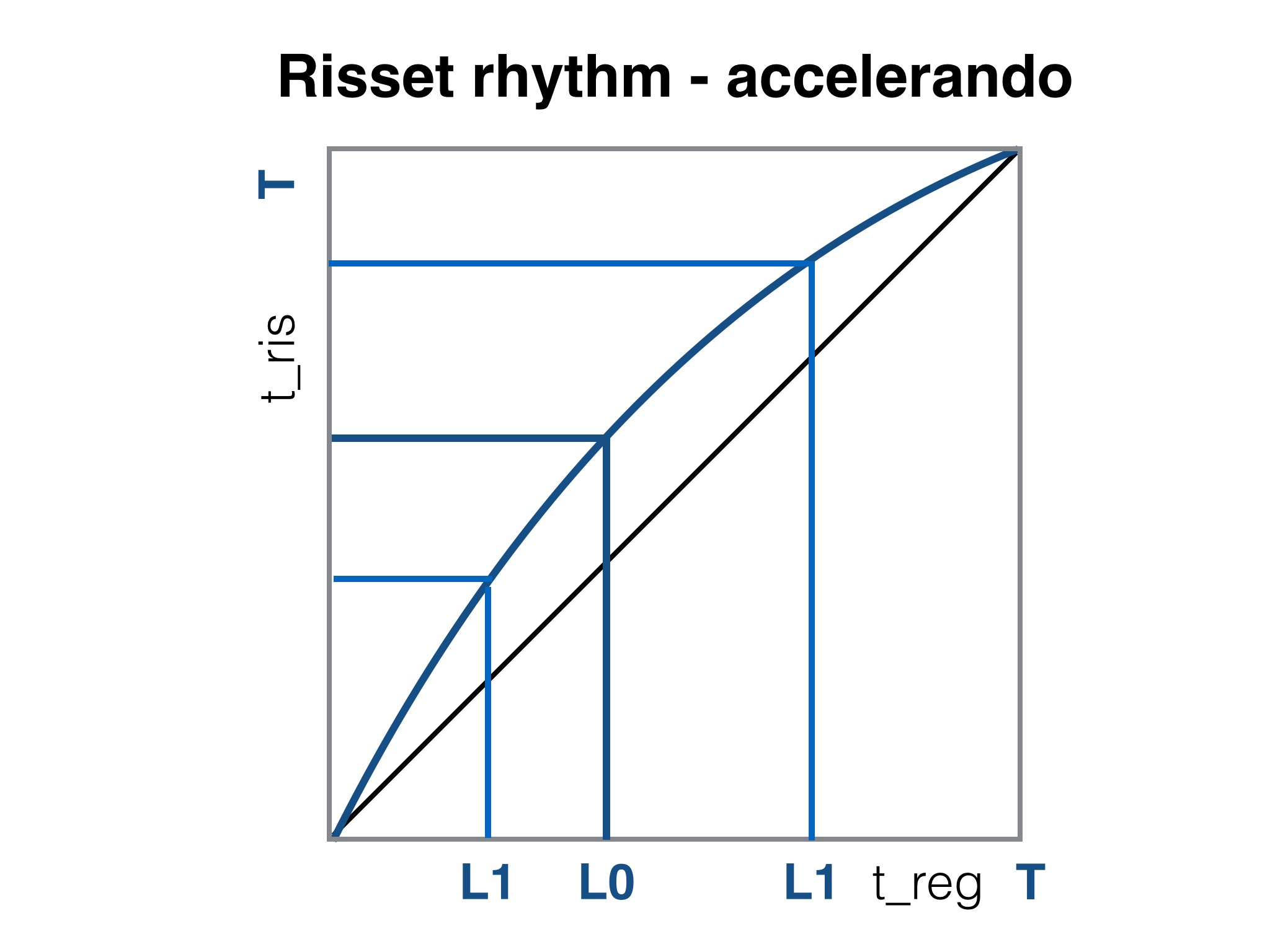Homepage > Documents > Music Toolkit - Risset rhythm MIDI tick calculator

# DAW Midi tick timing calculator for accelerating and decelerating Risset rhythms

## Risset rhythm MIDI tick calculator - Version 1.0This tool calculates the MIDI tick timing for accelerating and decelerating Risset rhythms. Select the Length of the pattern (number of measures), the Meter, the Division (smallest note value), the PPQ (MIDI tick resolution per quarter note) and the Mode (speeding, slowing). The tool will then Calculate the appropriate note positions in the sequencer MIDI editor at different rhythmic timescales.

Risset Rhythm Input Parameters
Number of measures: Meter [n1/n2]: 1 2 3 4 5 6 7 8 2 3 4 5 6 7 8 9   / 2 4 8 1 2 2t 4 4t 8 8t 16 16t 32 384 480 960 accelerando (speeding up) ritardando (slowing down) (Then click on a first column cell)
 Original Note Position Risset Rhythm Note Position MIDI position: M.b.t % of total length MIDI position: M.b.t % of total length

### Guidelines for the userThis JavaScript GUI provides a MIDI editor tick calculation tool for accelerating and decelerating Risset rhythms. Composer Jean-Claude Risset published and used these rhythms in electronic music. The technique also appears in Electronic Dance Music (EDM) productions. Over the Risset pattern length the tempo will double (accelerando) of halve (slowing down) along a logarithmic scale (logarithm to base 2, to be precise). These rhythms are repeated at smaller scales (the fractal, self-scaling property). For a set of input parameters the MIDI note position in the Risset rhythmic pattern will be calculated and displayed in a table. The figure on the right shows the logarithmic mapping of the pattern with length T with regular timing treg on the Risset accelerando rhythm time axis tris. The inverse of the blue curve slope is a measure for the instantaneous tempo. The mapping of the note at two rhythmic timescale levels, L0 and L1, is shown.

Use this GUI as follows:

• The original, regular rhythmic pattern consists of a Number of Measures. Use the radio button to select between 1 and 8 measures.
• Then select the Meter as a ratio of two integer numbers, e.g., 4/4 (four quarter notes in the measure). The first radio button selects the number of notes in the measure (values between 2 and 9), the second integer indicates the unit (half, quarter or 8th notes).
• Now pick the Note resolution, i.e., the smallest note value in the original rhythm pattern. The radio button lets you select between whole notes (value: 1) and 32th notes (value: 32), including triplet values (e.g., quarter note triplets, indicated as 4t).
• The sequencer software will use a certain MIDI tick resolution, i.e., a number or pulses per quarter note [PPQ]. The current standard value is the highest resolution of 960 ticks per quarter note (Logic Pro, Pro Tools, Cubase), but selecting a lower resolution is an option.
• Finally, choose the Risset rhythm Mode. The rhythm may be accelerating or slowing down (ritardando).
• Pressing the Start calculation button yields a table with MIDI note positions. The table shows the original, regular note positions in the first two columns. The first column contains the measure-beat-tick [M.b.t] values, the second column shows the position of the note as a percentage [%] of the total pattern duration. Column three and four show the Risset rhythm note positions, also as [M.b.t] and percentage values. E.g., a regular quarter note beat pattern in an accelerating Risset rhythm yields shorter time differences between the beats towards the pattern end.
• The values in column three also display the rhythmic positions at the higher timescale level (faster rhythm, shorter note values) of the Risset rhythm. To facilitate the reading of the different levels, click on a cell in the first column. When this note number is a multiple of 2, 4, or 8, the blue background colour shading in the third column highlights the equivalent note MIDI positions at multiple levels, starting at Level 0. There will be two coloured notes at timescale Level 1, four at Level 2 and eight at Level 3 (the current maximum).
• Press the Reset defaults button to reset the input variables to their default values. [TIP]: For irregular or mixed rhythms, rerun this tool at different resolutions (note divisions). Copy the appropriate MIDI tick values as [m.b.t.]-tuples on a piece of paper. Then enter the notes in the sequencer MIDI editor.

The recommended procedure for implementing Risset rhythms is:

1. Create a rhythmic pattern with length Nmeas on a regular timing grid in a meter (e.g., 4/4). This is the Level 0 (L0) original pattern.
2. Start the Risset rhythm calculation tool, and find the corresponding MIDI note timing [M.b.t] at timescale levels L0, L1, …, Ln. The length of the pattern at Level 1 is Nmeas /2, at Level 2 is Nmeas /4, etc. Usually n=2-3 levels will suffice for the rhythmic effect.
3. Enter the Risset rhythms at all levels L0 - Ln as regions/patterns in the sequencer MIDI editor. Assign (percussive) sounds to the rhythm. Copy the patterns on parallel tracks.
4. Apply a (1-cos) sound power level (SPL) curve over the timescale levels; Level 0 and Level n are at low SPL, maximum SPL at Level n/2. Then play the patterns and listen to the effect.

### Reference sources

Here's a list of references:

• Wikipedia, Jean-Claude Risset. URL http://en.wikipedia.org/wiki/Jean-Claude_Risset (visited May 2014).
• Dan Stowell, Scheduling and composing with Risset eternal accelerando rhythms. (PDF) Centre for Digital Music, Queen Mary University of London, UK (2011). URL: c4dm.eecs.qmul.ac.uk/papers/2011/Stowell2011icmc.pdf.